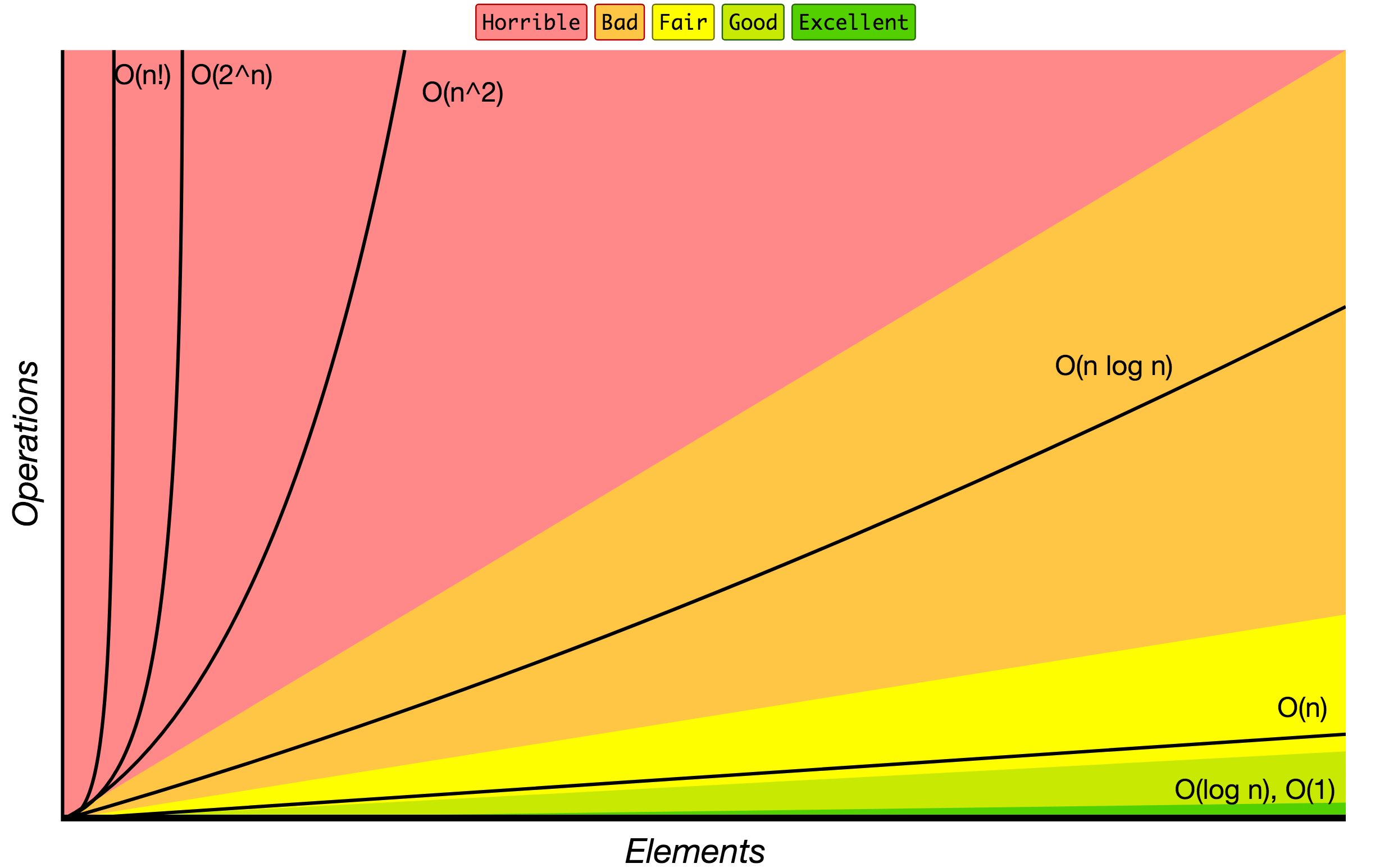# JavaScript Algorithms and Data Structures

This repository contains JavaScript based examples of many popular algorithms and data structures.

Each algorithm and data structure has its own separate README with related explanations and links for further reading (including ones to YouTube videos).

Read this in other languages: 简体中文, 繁體中文, 한국어, 日本語, Polski, Français, Español, Português, Русский, Türk, Italiana, Bahasa Indonesia, Українська, Arabic, Deutsch

☝ Note that this project is meant to be used for learning and researching purposes only, and it is not meant to be used for production.

## Data Structures

A data structure is a particular way of organizing and storing data in a computer so that it can be accessed and modified efficiently. More precisely, a data structure is a collection of data values, the relationships among them, and the functions or operations that can be applied to the data.

`B` - Beginner, `A` - Advanced

## Algorithms

An algorithm is an unambiguous specification of how to solve a class of problems. It is a set of rules that precisely define a sequence of operations.

`B` - Beginner, `A` - Advanced

### Algorithms by Topic

An algorithmic paradigm is a generic method or approach which underlies the design of a class of algorithms. It is an abstraction higher than the notion of an algorithm, just as an algorithm is an abstraction higher than a computer program.

## How to use this repository

Install all dependencies

``````npm install
``````

Run ESLint

You may want to run it to check code quality.

``````npm run lint
``````

Run all tests

``````npm test
``````

Run tests by name

``````npm test -- 'LinkedList'
``````

Troubleshooting

In case if linting or testing is failing try to delete the `node_modules` folder and re-install npm packages:

``````rm -rf ./node_modules
npm i
``````

Playground

You may play with data-structures and algorithms in `./src/playground/playground.js` file and write tests for it in `./src/playground/__test__/playground.test.js`.

Then just simply run the following command to test if your playground code works as expected:

``````npm test -- 'playground'
``````

## Useful Information

### References

▶ Data Structures and Algorithms on YouTube

### Big O Notation

Big O notation is used to classify algorithms according to how their running time or space requirements grow as the input size grows. On the chart below you may find most common orders of growth of algorithms specified in Big O notation.Source: Big O Cheat Sheet.

Below is the list of some of the most used Big O notations and their performance comparisons against different sizes of the input data.

Big O Notation Computations for 10 elements Computations for 100 elements Computations for 1000 elements
O(1) 1 1 1
O(log N) 3 6 9
O(N) 10 100 1000
O(N log N) 30 600 9000
O(N^2) 100 10000 1000000
O(2^N) 1024 1.26e+29 1.07e+301
O(N!) 3628800 9.3e+157 4.02e+2567

### Data Structure Operations Complexity

Data Structure Access Search Insertion Deletion Comments
Array 1 n n n
Stack n n 1 1
Queue n n 1 1
Linked List n n 1 n
Hash Table - n n n In case of perfect hash function costs would be O(1)
Binary Search Tree n n n n In case of balanced tree costs would be O(log(n))
B-Tree log(n) log(n) log(n) log(n)
Red-Black Tree log(n) log(n) log(n) log(n)
AVL Tree log(n) log(n) log(n) log(n)
Bloom Filter - 1 1 - False positives are possible while searching

### Array Sorting Algorithms Complexity

Name Best Average Worst Memory Stable Comments
Bubble sort n n2 n2 1 Yes
Insertion sort n n2 n2 1 Yes
Selection sort n2 n2 n2 1 No
Heap sort n log(n) n log(n) n log(n) 1 No
Merge sort n log(n) n log(n) n log(n) n Yes
Quick sort n log(n) n log(n) n2 log(n) No Quicksort is usually done in-place with O(log(n)) stack space
Shell sort n log(n) depends on gap sequence n (log(n))2 1 No
Counting sort n + r n + r n + r n + r Yes r - biggest number in array
Radix sort n * k n * k n * k n + k Yes k - length of longest key

## Project Backers

You may support this project via ❤️️ GitHub or ❤️️ Patreon.

Folks who are backing this project `∑ = 0`

ℹ️ A few more projects and articles about JavaScript and algorithms on trekhleb.dev

Get A Weekly Email With Trending Projects For These Topics
No Spam. Unsubscribe easily at any time.
Javascript (1,509,952
Algorithms (10,601
Data Structures (6,189
Computer Science (1,448
Interview (1,142
Interview Preparation (511
Related Projects